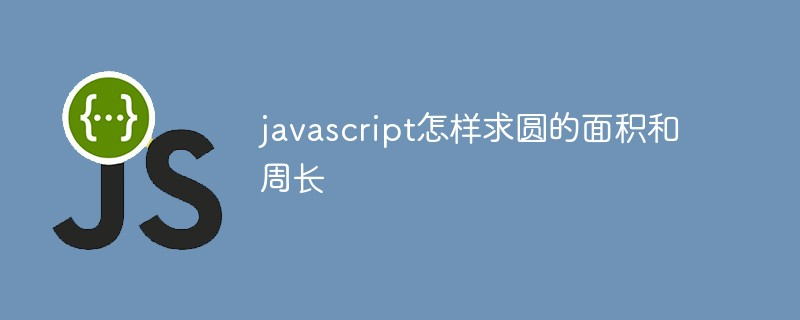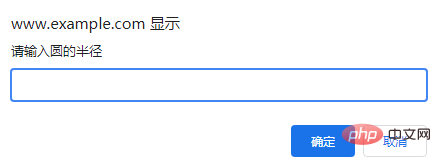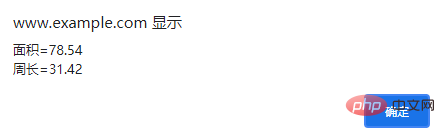# javascript怎样求圆的面积和周长javascript怎样求圆的面积和周长

Math 对象并不像 Date 和 String 那样是对象的类，因此没有构造函数 Math()。

```var x = Math.PI; // 返回PI
var y = Math.sqrt(16); // 返回16的平方根```

`Math.pow(x,y)`

pow() 方法返回 x 的 y 次幂。

```<!DOCTYPE html>
<html lang="en">
<meta charset="UTF-8">
<meta name="viewport" content="width=device-width, initial-scale=1.0">
<meta http-equiv="X-UA-Compatible" content="ie=edge">
<title>Document</title>
<body>
<script>
//四舍五入
function round(number, X) {
X = (!X ? 2 : X);
return Math.round(number*Math.pow(10,X))/Math.pow(10,X);
}
var r = Number(prompt('请输入圆的半径'));
if(!isNaN(r)) {
var m = round(Math.PI * Math.pow(r, 2), 2);
var c = round(2 * Math.PI * r);
alert('面积=' + m + '\n' + '周长=' + c);
} else {
}
</script>
</body>
</html>```【推荐学习：javascript高级教程### 全部评论我要评论

• 取消发布评论发送
• 1/1